# 玩转YY老师服务器(上)之IDA反编译伪代码还原插件编写

### 玩转YY老师服务器(上)之IDA反编译伪代码还原插件编写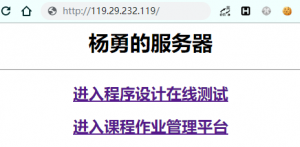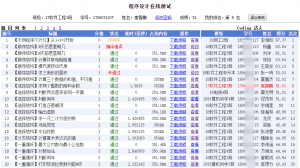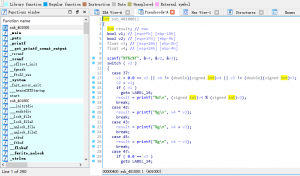https://www.hex-rays.com/products/ida/support/idapython_docs/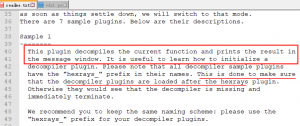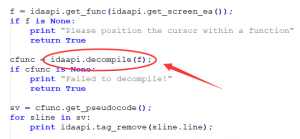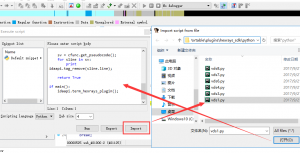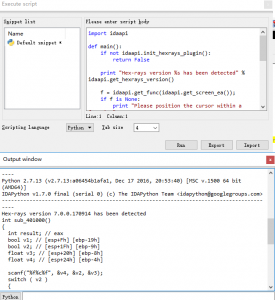```import re
import idaapi

Wait()

def dc(func):
func = idaapi.get_func(get_name_ea_simple(func))
return str(idaapi.decompile(func))

def search_sub(dec):
sub_name = dec
if isinstance(dec,str):
dec = dc(dec)
try:
use = re.findall('sub_[0-9A-F]{6}',dec)
for x in use:
if sub_name == x:
continue
if x not in sub_use:
sub_use.append(x)
search_sub(x)
else:
continue
except Exception as e:
print e

def rep(code):
r_dict = {'__cdecl':'','__thiscall':'','__fastcall':'','BOOL':'bool','printf("\n");\n':'',
'int argc, const char **argv, const char **envp':'','LODWORD':'int',
'system("pause");\n':'','_DWORD':'unsigned long'}
for i in r_dict.items():
code = code.replace(i,i)
return code

sub_use = ['_main']
search_sub('_main')
code_list = map(dc,sub_use)
code_list = map(rep,code_list)
output = GetInputFile().split('.')+'.cpp'

f = open(output,'a')
f.write('#include&lt;{}&gt;\n'.format(i))
for i in code_list[::-1]:
f.write('\n'+i+'\n')
f.close()
print 'decompile success!'
#Exit(0)```

Wait()

get_name_ea_simple():是根据函数名获取函数地址

idaapi.get_func(): 这个函数来获取函数的边界地址(起始和结束地址)

idaapi.decompile():就是得到伪代码的函数

GetInputFile():获取当前反编译文件的文件名

dc是一个由函数名得到伪代码的函数。rep本质就是一个替换函数，因为ida中一些关键字拿给C++编译器是无法识别的，所以我用了字典来实现键值对即原值和替换值得对应，再遍历这个字典实现replace。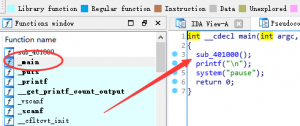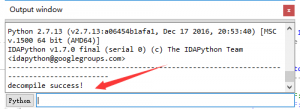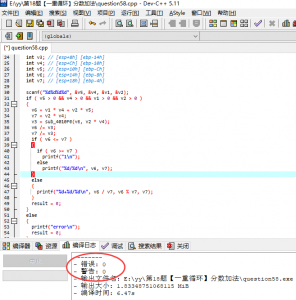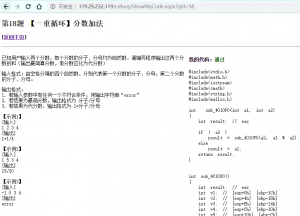微信赞赏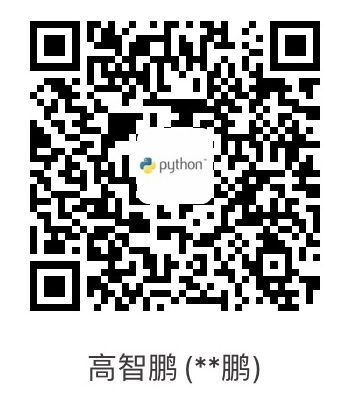支付宝赞赏